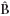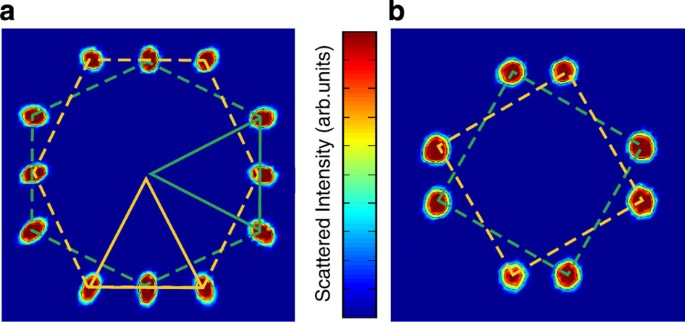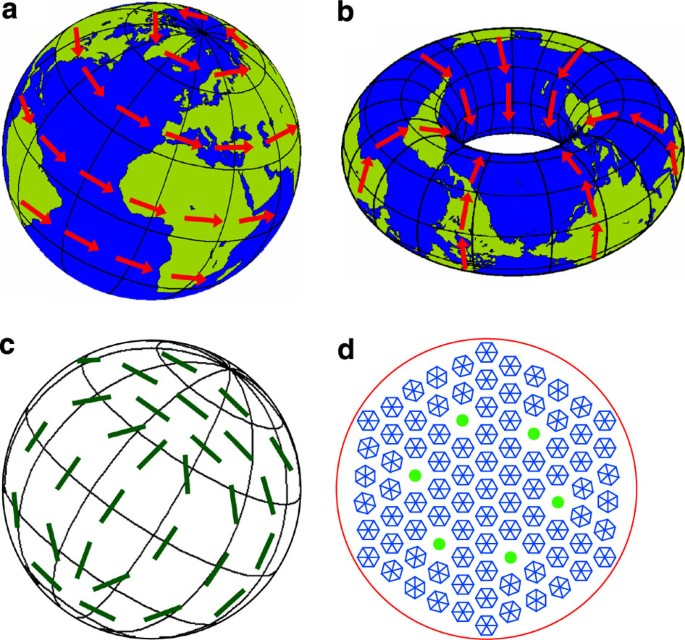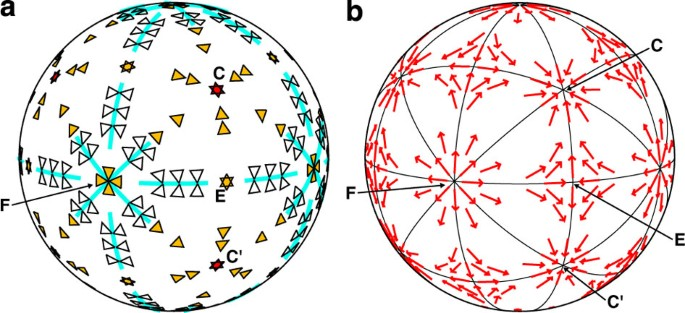Thank you for visiting nature.com. You are using a browser version with limited support for CSS. To obtain the best experience, we recommend you use a more up to date browser (or turn off compatibility mode in Internet Explorer). In the meantime, to ensure continued support, we are displaying the site without styles and JavaScript.

# Magnetic flux lines in type-II superconductors and the 'hairy ball' theorem

## Abstract

Many prominent phenomena originate from geometrical effects rather than from local physics. For example, the 'hairy ball' (HB) theorem asserts that a hairy sphere cannot be combed without introducing at least one singularity, and is fulfilled by the atmospheric circulation with the existence of stratospheric polar vortices and the fact that there is always at least one place on Earth where the horizontal wind is still. In this study, we examine the consequences of the HB theorem for the lattice of flux lines that form when a magnetic field is applied to a type-II superconducting crystal. We find that discontinuities must exist in lattice shape as a function of field direction relative to the crystal. Extraordinary, 'unconventional' flux line lattice shapes that spontaneously break the underlying crystal symmetry are thus remarkably likely across all type-II superconductors, both conventional and unconventional.

## Introduction

A type-II superconductor responds to a moderate magnetic field by admitting the field as quantised lines1, each carrying flux Φ0=h/2e. These lines nominally arrange in a two-dimensional lattice, with one Φ0 per unit cell2. An equilateral triangular half-unit cell is expected in the isotropic case, but other shapes are frequently observed3,4,5,6,7,8,9,10,11 and are usually interpreted in terms of anisotropies of the crystal or of the superconducting wave function. Hence, it is not surprising that, as one of the few probes of the superconducting state, the study of flux line lattice (FLL) shapes and orientations forms a cornerstone in our understanding of both conventional and unconventional superconductivity. In this study, we reveal that the occurrence of unusual FLL structures is rather likely for purely geometrical reasons, because the FLL must comply with the 'hairy ball' (HB) theorem12,13. We illustrate the remarkable, different ways in which this geometrical constraint may be fulfilled by considering the particular case of a superconducting niobium (Nb) crystal, though our arguments are general. For any superconductor, tuning the field value and temperature and varying the field direction may 'comb the HB' in a variety of different 'hairstyles'.

The FLL may be imaged directly by neutron diffraction (Fig. 1): the positions of the diffraction spots relative to the incoming beam give the lattice vectors (see Methods). Within the superconductor, flux lines run parallel to the magnetic induction B. For a given B=|B|, the directionsestablish a sphere, and the lattice vectors of the FLL, tangent to the sphere, can be envisaged as 'hairs'. The HB theorem states that a continuous non-vanishing tangent vector cannot exist everywhere on a sphere. The original proof arises from the Poincaré–Hopf theorem12,13.Figure 1: Diffraction patterns from flux line lattices (FLLs) formed in niobium.

The lattice vectors describing the two-dimensional FLL lie perpendicular to the direction of magnetic induction, and by itself each lattice vector must satisfy the HB theorem. In this study, we consider the FLL shape formed by two of these lattice vectors and find that this cannot vary smoothly with field direction. We further examine the nature of the singularities in FLL shape, which appear intriguingly different from those of a tangent vector.

## Results

### Singularities in FLL structure

The Poincaré-Hopf theorem states that the total 'winding number' of a vector field on a surface is equal to χ, the 'Euler characteristic' of that surface12,13. We remind the reader that for a polyhedron, χ is the number of faces minus the number of edges plus the number of vertices; for a cube, χ=6−12+8=2 and it is homeomorphic to a sphere; hence, χ=2 for a sphere also. This means that a vector field on its surface must circulate around at least one singularity.

In Figure 2a, we illustrate a singularity with winding number w=+1. The value of w reflects the fact that on going around the singularity, the vector direction rotates by one full turn. The HB theorem can be satisfied on a sphere by the existence of two of these singularities, for example, westerly winds circulating around the globe except for still points at the North and South poles (Fig. 2a). The inevitable existence of singularities on a sphere, which affects the physics on a global scale, does not originate from the local physics, but arises from underlying geometry. For example, if the Earth were toroidal (χ=0), winds could circulate in the same local direction everywhere (Fig. 2b).Figure 2: Surface patterns of tangent objects and the hairy ball theorem.

In atmospheric physics, singularities may be realised by making the tangent vector—the wind velocity—vanish, as at the eye of a storm. In contrast, null FLL vectors are forbidden by flux quantisation, which fixes the FLL unit cell area A=Φ0/B. In turn, this guarantees that the pair of basis vectors describing the FLL are linearly independent. The maximal number m of continuous linearly independent vector fields that a sphere in n dimensions can support is assessable analytically14,15,16,17. For n odd, it is found that m=0, consistent with the HB theorem for n=3. As neither of the two FLL basis vectors can be continuous, the FLL shape cannot vary smoothly with field direction.

Tangent vectors and FLL shapes are clearly rather different objects, and indeed, these differences are reflected in the distinctive nature of their singularities. However, the concept of 'winding number' is preserved. For tangent vectors, w must be integral (see Fig. 2a), but tangent objects of higher symmetry can adopt a fractional w. In Figure 2c, we illustrate half-integral winding number singularities for a twofold symmetric object: a vector without an arrow. The Euler characteristic χ depends only on the surface and remains +2 for a sphere. Thus, to 'comb a ball of uprooted hairs', four w=+½ singularities are a possible solution. In Figure 2d, we consider tangent objects having sixfold symmetry, appropriate for an equilateral triangular FLL. Here, a w=+1 singularity can be 'delocalised' into six w=+1/61/6 points. Remarkably, we shall see that such singularities actually exist—in equilibrium—in nature.

There are several ways by which the FLL structure can satisfy the HB theorem, one or more of which may occur in any given type-II superconductor. We examine three ways that are observed experimentally.

### No favoured FLL orientation

The first way is trivial: no particular FLL orientation is favoured. As FLL orientation is not determined by standard approaches1,2 based on 'conventional' (s-wave) theory18, underlying anisotropy specific to the superconductor, even if weak, is a prerequisite to establishing any preferred FLL orientation, even when the FLL shape is a 'conventional' equilateral triangle. Any impurities present will smooth out underlying anisotropy19 and also pin the FLL. Therefore, it is unsurprising that observations of orientationally disordered FLL's have been the preserve of situations in which impurity or pinning effects are significant. Examples include 2H-NbSe220,21, Bi2Sr2CaCu2O822, the nickel borocarbide superconductors10,11 and iron pnictides23, as well as experiments in which strong disorder is purposely introduced24.

### FLL domain formation

A second way may occur when the field lies along a symmetry direction or in a symmetry plane of the superconducting crystal. Two or more degenerate FLL structures can then exist, all with the same shape but orientated differently, as permitted by the crystal symmetries. FLL domains with any one of these degenerate orientations will form in different parts of the crystal. On rotatingthrough the symmetry position, the domain occupations will change rapidly, such that one set of FLL vectors will fade in diffracted neutron intensity, simultaneously being replaced by a differently orientated set. This is exemplified by the FLL shapes and orientations observed in high-purity Nb, a conventional, low-κ superconductor with cubic crystal structure3,4,5,6. In Figure 1a, we show the FLL observed at high temperatures for fields parallel to  (see Methods). Here, the FLL half-unit cell is an isosceles triangle. This twofold shape spontaneously breaks the fourfold crystal symmetry, and two degenerate FLL domains co-exist.

To understand how spontaneous symmetry breaking and domain formation help satisfy the HB theorem, we compare in Figure 3 the FLL shapes and orientations observed in Nb as a function of field direction (Fig. 3a), with the simplest configuration of tangent vectors on a sphere possessing cubic symmetry (Fig. 3b). In Figure 3b, w=+1 singularities are placed at the six cube-face (labelled 'F') axes having fourfold symmetry and also at the eight cube-corner ('C') threefold directions, whereas w=−1 singularities are placed at the 12 twofold cube-edge ('E') directions. Comparing with Figure 3a, we see that the FLL shape keeps its continuity for field directions near C by effectively 'delocalising' the singularity (see Fig. 2d). At E, the singularity is also delocalised: by following the FLL shape asrotates in the CEC′ mirror plane, a geometric phase shift of π is seen between the equilateral triangular half-unit cells originating at C and C′, yet at E, both half-unit cells represent the same isosceles FLL. In stark contrast, a local character remains at the fourfold axis F, where two line singularities along the FC and FC′ directions intersect (see Fig. 3a). At F, smooth distortions in FLL shape are afforded through the coexistence of multiple FLL domains of similar shape but different orientation. We point out that the FLL's geometric phase twists discontinuously as the F point is circumnavigated; rapid switches in domain occupancy are observed ascrosses the FC and FC' mirror planes5.Figure 3: Flux line lattice (FLL) structures and the hairy cube.

### Jumps in FLL shape

A third way to realise the HB theorem is by discontinuous changes in FLL shape with field direction. This behaviour is also exhibited by high-purity Nb at low temperatures (≤ 2.5 K) and fields within the intermediate mixed state. Unlike in the mixed state, in which the sample is packed with flux lines, in the intermediate mixed state, the sample contains Meissner regions of zero flux and regions of flux lines25,26. Atparallel to  in Nb, the unit FLL cell is exactly square, but its orientation breaks the fourfold crystal symmetry, and two degenerate domains coexist (Fig. 1b). This square shape is observed to transform discontinuously with rotations ofaway from 6. Unlike in the mixed state in which flux lines interact repulsively, in the intermediate mixed state, the interaction is attractive over a range of distances25. It would be intriguing to determine if this change in local physics affects whether the HB theorem is satisfied by the appearance of discontinuous distortions or whether it is relieved through FLL domain formation.

## Discussion

We conclude that spontaneous symmetry-breaking FLL structures are surprisingly likely to occur. If the FLL elects to fulfill the HB theorem by forming domains, then domain formation implies degenerate FLL orientations, which compel the FLL structure to break some underlying crystal symmetry. FLL's are sometimes dubbed 'unconventional' if they depart from the equilateral triangular prediction of standard Ginzburg–Landau theory2, valid for conventional (s-wave) isotropic superconductors18. The HB theorem makes it clear that 'unconventional' FLL structures are not exclusive to unconventional superconductors and therefore do not necessarily imply unconventional superconductivity.

Although geometry decrees that the FLL must cleave to the HB theorem, it is local physics that determines exactly how this is achieved. Clearly, the HB theorem will be seminal in stimulating FLL explorations as a function of field direction. To date, a survey of symmetry-breaking FLL's has been reported only in Nb3,4,5,6, in which intriguing FLL structures and transitions are observed at so-called 'magic'directions away from the principal crystal axes. These 'magic' directions are likely tied to corresponding features in the anisotropic Nb Fermi surface5,6.

The additional asymmetries introduced by unconventional superconducting wave functions may allow new local physics to emerge. We point out that PrOs4Sb12 is an alluring candidate for prospective angle-resolved FLL morphology studies. With tetrahedral (Th) point group symmetry and no four-fold rotation axes, PrOs4Sb12 reveals a uniquely orientated, distorted triangular FLL for fields parallel to one of the a, b or c axes27. The HB theorem stipulates that interesting FLL transitions must occur as the field is rotated; these transitions may provide valuable information as to the hitherto unresolved nature of the PrOs4Sb12 superconducting gap. Finally, we remark that it will be fascinating to ascertain which of the distinct modes are exhibited and the location of singularities on the-rotation sphere, not only in conventional and unconventional superconductors but also in any structure featuring one or more ordering vectors normal to B, such as the magnetic 'skyrmion lattice' in MnSi28.

## Methods

### Small-angle neutron scattering

The FLL structures of high-purity Nb, presented in this paper to illustrate the HB theorem, were measured using a double-crystal diffractometer at Oak Ridge National Laboratory3 and small-angle neutron scattering (SANS) instruments at the Institut Laue-Langevin and the Paul Scherrer Institut5. In a typical SANS setup, neutrons of wavelengths ≈10 Å were collimated over a distance ≈18 m before reaching the sample. A two-dimensional area detector at a similar distance behind the sample detected the diffracted neutrons. The samples—large (≈10 g), high-purity single crystals of Nb—were mounted inside a cryomagnet providing a magnetic field approximately parallel to the incident neutron beam. A full description of the SANS measurements and sample details are contained in Laver et al.5, and the double-crystal diffractometer measurements are described in Christen et al.3

The FLL diffraction patterns shown in Figure 1 depict integrated intensities, collected by rocking the Bragg diffraction spots through the Ewald sphere. As the FLL is two-dimensional, the real space arrangement is readily visualised from the reciprocal lattice vectors by rotating these diffraction images by 90° about the field axis (normal to the page in Fig. 1).

Elemental Nb is bcc and has a low Ginzburg–Landau parameter (κ0.77) near Tc=9.27 K. With fields B parallel to the four-fold  crystal axis, a cornucopia of distinct FLL structures is observed, depending on the temperature and field value4. In contrast, at all temperatures and fields parallel to the three-fold  crystal axis, a single domain of equilateral triangular FLL is observed3,5. In this paper, we pick two regions of temperature and field value that aptly show the different ways in which the HB theorem may manifest itself as the field directionis rotated relative to the crystal. The conditions for the first region are high temperatures (≤4 K); at all values of field parallel to , the FLL half-unit cell is an isosceles triangle, and two degenerate FLL domains coexist (Fig. 1a). The observed evolution of this FLL as the field is rotated is shown in Figure 3a. This figure is compiled from results unveiled in Christen et al.3 and Laver et al.5 For the second region, the conditions are low temperatures (≤2.5 K) and fields within the intermediate mixed state. The intermediate mixed state arises in superconductors of low κ close to 1√2, at field values close to the lower critical field and in samples with a finite demagnetisation factor25,26. Under these conditions and withparallel to , a square unit cell is observed with nearest-neighbour directions ≈ ± 15° from the <100> crystal directions, as shown in Figure 1b. The observed evolution with field directionof this FLL is detailed in Mühlbauer et al.6

How to cite this article: Laver, M. et al. Magnetic flux lines in type-II superconductors and the 'hairy ball' theorem. Nat. Commun. 1:45 doi: 10.1038/ncomms1047 (2010).

## References

1. 1

London, F. Superfluids (Wiley, 1950).

2. 2

Abrikosov, A. A. On the magnetic properties of superconductors of the second group. Sov. Phys. JETP 5, 1174–1183 (1957).

3. 3

Christen, D. K., Kerchner, H. R., Sekula, S. T. & Thorel, P. Equilibrium properties of the fluxoid lattice in single-crystal niobium. II. Small-angle neutron-diffraction measurements. Phys. Rev. B 21, 102–117 (1980).

4. 4

Laver, M. et al. Spontaneous symmetry-breaking vortex lattice transitions in pure niobium. Phys. Rev. Lett. 96, 167002 (2006).

5. 5

Laver, M. et al. Structure and degeneracy of vortex lattice domains in pure superconducting niobium. Phys. Rev. B 79, 014518 (2009).

6. 6

Mühlbauer, S. et al. Morphology of the superconducting vortex lattice in ultrapure niobium. Phys. Rev. Lett. 102, 136408 (2009).

7. 7

Riseman, T. M. et al. Observation of a square flux-line lattice in the unconventional superconductor Sr2RuO4 . Nature 396, 242–245 (1998).

8. 8

Huxley, A. et al. Realignment of the flux-line lattice by a change in the symmetry of the superconductivity in UPt3 . Nature 406, 160–164 (2000).

9. 9

Gilardi, R. et al. Direct evidence for an intrinsic square vortex lattice in the overdoped high-T c superconductor La1.83Sr0.17CuO4+δ . Phys. Rev. Lett. 88, 217003 (2002).

10. 10

Eskildsen, M. R. et al. Observation of a field-driven structural phase transition in the flux line lattice in ErNi2B2C. Phys. Rev. Lett. 78, 1968–1971 (1997).

11. 11

Eskildsen, M. R. et al. Structural stability of the square flux line lattice in YNi2B2C and LuNi2B2C studied with small-angle neutron scattering. Phys. Rev. Lett. 79, 487–490 (1997).

12. 12

Poincaré, H. Sur les courbes définies par les équations différentielles. J. Math. Pures Appl. 4, 167–244 (1885).

13. 13

Hopf, H. Abbildungsklassen n-dimensionaler Mannigfaltigkeiten. Math. Ann. 96, 209–224 (1927).

14. 14

Hurwitz, A. Über die Komposition der quadratischen Formen. Math. Ann. 88, 1–25 (1923).

15. 15

Radon, J. Lineare Scharen orthogonaler Matrizen. Abh. Math. Sem. Univ. Hamburg I, 1–14 (1923).

16. 16

Eckmann, B. Gruppentheoretischer Beweis des Satzes von Hurwitz-Radon über der Komposition quadratischer Formen. Comment. Math. Helv. 15, 358–366 (1943).

17. 17

Adams, J. F. Vector fields on spheres. Ann. Math. 75, 603–632 (1962).

18. 18

Bardeen, J., Cooper, L. N. & Schrieffer, J. R. Theory of superconductivity. Phys. Rev. 108, 1175–1204 (1957).

19. 19

Klein, U. et al. Microscopic calculations on the vortex state of Type-II superconductors. J. Low Temp. Phys. 69, 1–37 (1987).

20. 20

Bolle, C. A., De La Cruz, F., Gammel, P. L., Waszczak, J. V. & Bishop, D. J. Observation of tilt induced orientational order in the magnetic flux lattice in 2H-NbSe2 . Phys. Rev. Lett. 71, 4039–4042 (1993).

21. 21

Yaron, U. et al. Neutron diffraction studies of flowing and pinned magnetic flux lattices in 2H-NbSe2 . Phys. Rev. Lett. 73, 2748–2751 (1994).

22. 22

Grier, D. G. et al. Translational and bond-orientational order in the vortex lattice of the high-T c superconductor Bi2.1Sr1.9Ca0.9Cu2O8+δ . Phys. Rev. Lett. 66, 2270–2273 (1991).

23. 23

Eskildsen, M. R. et al. Vortices in superconducting Ba(Fe0.93Co0.07)2As2 studied via small-angle neutron scattering and Bitter decoration. Phys. Rev. B 79, 100501 (2009).

24. 24

Fasano, Y. et al. Observation of periodic vortex pinning induced by Bitter decoration. Phys. Rev. B 60, R15047–R15050 (1999).

25. 25

Kramer, L. Interaction of vortices in type II superconductors and the behavior near H c1 at arbitrary temperature. Z. Phys. 258, 367–380 (1973).

26. 26

Leung, M. C. Attractive interaction between vortices in type-II superconductors at arbitrary temperatures. J. Low Temp. Phys. 12, 215–235 (1973).

27. 27

Huxley, A. D. et al. Flux-line lattice distortion in PrOs4Sb12 . Phys. Rev. Lett. 93, 187005 (2004).

28. 28

Mühlbauer, S. et al. Skyrmion lattice in a chiral magnet. Science 323, 915–919 (2009).

## Acknowledgements

We are grateful to E. Blackburn, L. Mejlbro and J.S. White for suggestions and comments. M.L. was supported by DanScatt, E.M.F. was supported in part by EPSRC Grant EP-G027161-1.

## Author information

Authors

### Contributions

E.M.F. and M.L. both developed the physical interpretation of the HB theorem for FLL's in superconductors.

### Corresponding author

Correspondence to Mark Laver.

## Ethics declarations

### Competing interests

The authors declare no competing financial interests.

## Rights and permissions

Reprints and Permissions

Laver, M., Forgan, E. Magnetic flux lines in type-II superconductors and the 'hairy ball' theorem. Nat Commun 1, 45 (2010). https://doi.org/10.1038/ncomms1047

• Accepted:

• Published:

• ### Geometry-induced topological superconductivity

• Po-Hao Chou
• , Chia-Hsin Chen
• , Shih-Wei Liu
• , Chung-Hou Chung
•  & Chung-Yu Mou

Physical Review B (2021)

• ### Topology of eeg wave fronts

• Arturo Tozzi
• , Edward Bormashenko
•  & Norbert Jausovec

Cognitive Neurodynamics (2021)

• ### Electrically induced droplet ejection dynamics under shear flow

• K. Ashoke Raman
• , Yi Sui

Physics of Fluids (2020)

• ### Projective mechanisms subtending real world phenomena wipe away cause effect relationships

• Arturo Tozzi
•  & David Papo

Progress in Biophysics and Molecular Biology (2020)

• ### Rotation of the magnetic vortex lattice in Ru7B3 driven by the effects of broken time-reversal and inversion symmetry

• A. S. Cameron
• , Y. S. Yerin
• , Y. V. Tymoshenko
• , P. Y. Portnichenko
• , A. S. Sukhanov
• , M. Ciomaga Hatnean
• , D. Mc K. Paul
• , G. Balakrishnan
• , R. Cubitt
• , A. Heinemann
•  & D. S. Inosov

Physical Review B (2019)